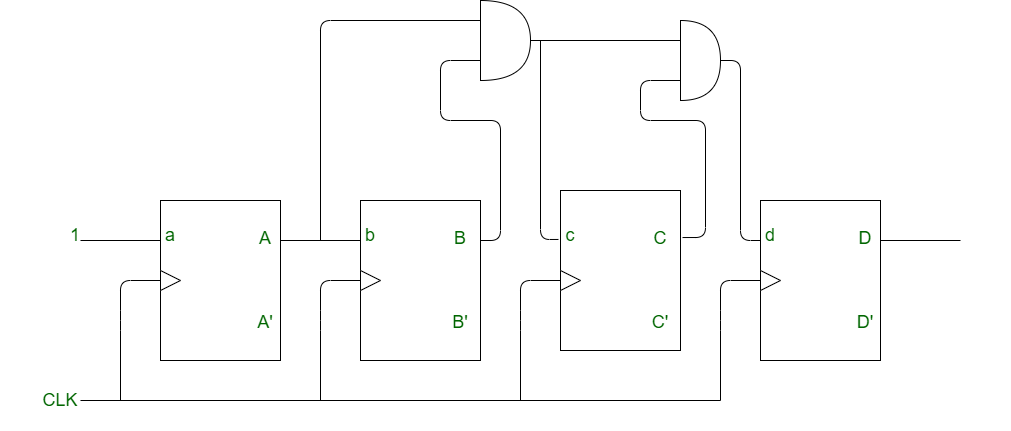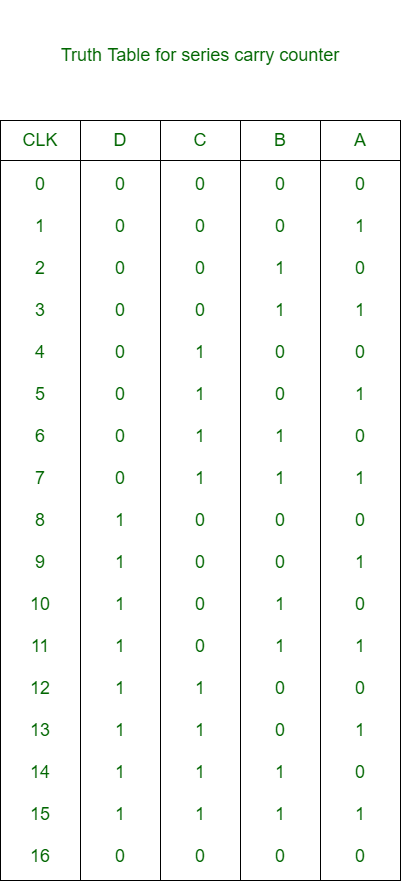Related Articles

# Synchronous Series Carry Counter

• Last Updated : 23 Apr, 2020

Synchronous Series Carry Counter is such a synchronous counter where inputs of flip-flops are connected in such a manner that only those flip-flops that are toggle on a given clock will have input value as the logic 1. The advantage of this counter is that it reduces the decoding error.

Block Diagram of Synchronous Series Carry Counter:
Here is the block diagram of a 4-bit (MOD-16) series carry counter.In the above counter:

1. A toggles for every CLK pulse is applied.
2. B toggles when A = 1 and CLK pulse is applied.
3. C toggles when B = 1 and A = 1 and CLK pulse is applied.
4. D toggles when C = B = A = 1 and CLK pulse is applied.

Truth Table for Series Carry Counter:Note:
In order to get the synchronous down counter, complemented output (A’, B’ etc.) of each stage is connected to the input of the next stage.

Total Time Delay :
The total delay for synchronous series carry counter is:

`T >= t(pd) + (n-2) x t(pd of AND gate) `

As the number of bit increases, the propagation delay of flip-flops and the propagation delay of AND gate also increases, this will increase the total delay of the circuit.